Sinusoids Next  |  Prev  |  Up  |  Top  |  Index  |  JOS Index  |  JOS Pubs  |  JOS Home  |  Search

## Sinusoids

A sinusoid is any function having the following form: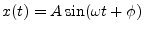where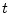is the independent (real) variable, and the fixed parameters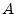,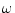, and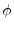are all real constants. In audio applications we typically have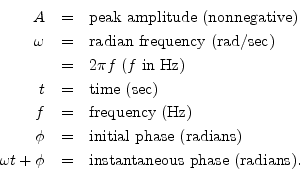An example is plotted in Fig.4.1.

The term peak amplitude'' is often shortened to amplitude,'' e.g., the amplitude of the tone was measured to be 5 Pascals.'' Strictly speaking, however, the amplitude of a signal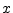is its instantaneous value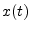at any time. The peak amplitudesatisfies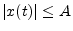. The instantaneous magnitude'' or simply magnitude'' of a signalis given by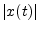, and the peak magnitude is the same thing as the peak amplitude.

The phase'' of a sinusoid normally means the initial phase'', but in some contexts it might mean instantaneous phase'', so be careful. Another term for initial phase is phase offset.

Note that Hz is an abbreviation for Hertz which physically means cycles per second. You might also encounter the notation cps (or c.p.s.'') for cycles per second (still in use by physicists and formerly used by engineers as well).

Since the sine function is periodic with period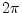, the initial phase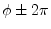is indistinguishable from. As a result, we may restrict the range ofto any lengthinterval. When needed, we will choose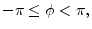i.e.,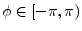. You may also encounter the convention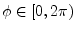.

Note that the radian frequencyis equal to the time derivative of the instantaneous phase of the sinusoid: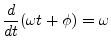This is also how the instantaneous frequency is defined when the phase is time varying. Let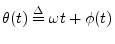denote the instantaneous phase of a sinusoid with a time-varying phase-offset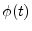. Then the instantaneous frequency is again given by the time derivative of the instantaneous phase: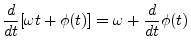Subsections
Next  |  Prev  |  Up  |  Top  |  Index  |  JOS Index  |  JOS Pubs  |  JOS Home  |  Search

[How to cite this work] [Order a printed hardcopy]